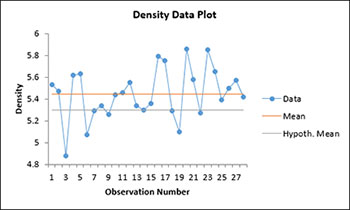# One Sample z and t Tests for a Mean HelpThis test is performed on the mean of one sample.  The test gives you a confidence interval for the mean and has the option to test the hypothesis that the mean is equal to, less than, or greater than a specified mean.  You have the following two options:

• t test: This test uses the t distribution and is usually used when with smaller sample sizes although it can be used with any sample size; this test uses the standard deviation calculated from the data.
• z test: This test uses the z distribution and is usually used with larger sample sizes (> 30) or if the standard deviation is known.

You also have the option for a two-sided, lower one-sided or an upper one-sided test.

The data can be in a worksheet or the user can enter an average, standard deviation, and sample size for the test.  The example below demonstrates how to do this test using data in a worksheet.

1. Enter the data into a worksheet as shown below.  The data can be one column, one row, or in multiple rows/columns.  The data can be downloaded at this link.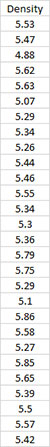2. Select the data above (including the heading).

3. Select “Sample Tests” from the “Statistical Tools” panel in the SPC for Excel ribbon.

4. Select the “One Sample z and t Tests for a Mean” option from the “Sample Tests” form.  Select OK and the input form below is displayed.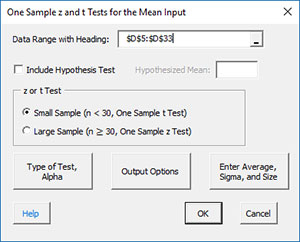• Data Range with Heading: This is the worksheet range containing the data and heading; the default is the selected worksheet range.
• Include Hypothesis Test: this option will include a hypothesis test in addition to the confidence interval. If selected, you must enter a hypothesized mean.
• Small or Large Sample Case:  this determines the case to use for the calculations; the default is based on the sample size.
• If the size is less than 30, the small sample case is selected. This will apply the t test.
• If the size is greater than or equal to 30 the large sample cause is selected.
• You may override this selection by selecting which case you want to use.
• Type of Test, Alpha: the input form below is displayed if this option is selected.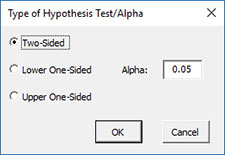• Select the Type of Test: two-sided; lower one-sided, or upper one-sided, the default is two-sided.
• Alpha: this is the confidence level; 1-alpha is the confidence interval, default is 0.05 or 95% confidence interval.
• Output Options: the input form below is displayed if this option is selected.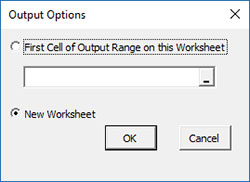• First Cell of Output Range on this Worksheet: Select this option if you want the output on an existing worksheet; then select the first cell of the range where you want the output placed; a check is made to ensure that no existing data are overwritten in the worksheet.
• New Worksheet: Select this option if you want the results on a new worksheet.
• Enter Average, Sigma and Size: the input form below is displayed if this option is selected.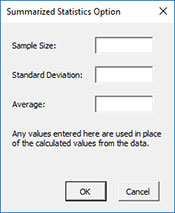• You many enter the sample size, standard deviation, or the average; any value that is entered will be used in place of the values calculated from the data.
• Select OK to generate the results.
• Select Cancel to end the program.

One Sample Test Output

The output from the example data (using the t test) is shown below.  The hypothesized mean is 5.3 in this example.   An explanation of terms is given below the output.  In addition to the output table, the program displays a chart showing the results.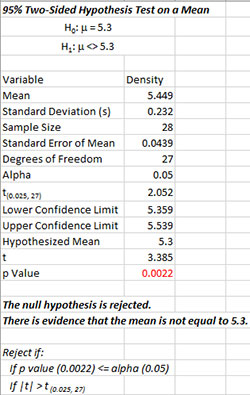The output tells you the conclusion from the test.  The null hypothesis (H0) and the alternate hypothesis (H1) are printed below the title.

• Variable: Name of variable
• Mean: Calculated average of data
• Standard Deviation (s): Calculated standard deviation
• Sample Size: Number of data points
• Standard Error of Mean: Calculated as standard deviation/sqrt(sample size)
• Degrees of Freedom: Calculated degrees of freedom = n-1
• Alpha: Value of alpha entered by user
• t(0.025, 28): t value based on alpha and degrees of freedom
• Lower Confidence Limit: Calculated lower confidence interval
• Upper Confidence Limit: Calculated upper confidence interval
• Hypothesized Mean: Specified mean entered by user
• t: Calculated t value
• p Value: Calculated p value (in red if p value ? alpha)

Notes:

• If the hypothesis test option is not selected, the output below the Upper Confidence Limit is not included.
• If the large sample size is selected, the z distribution will be used instead of the t distribution; the t in the output above will be replaced by z and the results will reflect the z distribution.
• If the average, sigma or sample size are entered, the print out will show these “entered.”
• If the option for a one-sided test is selected, there is only one confidence limit given.

The chart below is also created.  This makes it easy to see if the hypothesized mean is within or how far away it is from the confidence interval.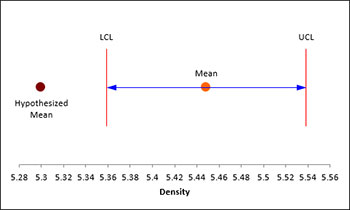There is also a chart of the data to look for possible outliers.  If the program detects possible outliers, they will be in red and a message will be printed on the worksheet.